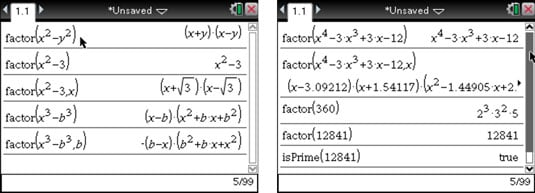##### TI-Nspire For Dummies, 2nd EditionThe Factor command from the TI-Nspire CAS Algebra submenu factors numerical and algebraic expressions. Choose [MENU]→Algebra→Factor to open the Factor command. Take a look at the first two lines of the first screen. TI-Nspire CAS attempts to factor any expression as much as possible with linear, rational, and real factors. The expression shown in the second line is not factorable based on these conditions.Notice in the third and fourth lines a comma is followed by a variable. By specifying a variable, TI-Nspire CAS produces linear factors (that is, factors in which the degree of the specified variable is 1) as long as these factors contain only real numbers.

The fourth and fifth lines are intended to illustrate that TI-Nspire CAS provides some sorting when it does its factoring. For example, notice that the fourth line is sorted with respect to the variable x (sorted means that x shows up first in the factors).

The fifth line specifies that you want to factor with respect to the variable b. As a result, the factors in the result lead with this variable.

Now, look at the second screen. The first line returns the original expression because the polynomial x4 – 3x3 + 3x – 12 is prime, meaning that it cannot be factored into linear, rational factors. However, by specifying that you want to factor in terms of the variable x (as shown in the second line), you make TI-Nspire produce linear factors with decimal approximations of irrational numbers, which provides a nice way to find the zeroes of a polynomial.

Finally, take a look at the third line of this screen, which illustrates that the Factor command factors numbers, too. The fourth line gives an example of what you get when you try to factor a prime number.

The computing time required to factor large composite numbers can be quite long. If you are interested only in determining whether a number is prime or composite, try using the isPrime command. This command returns the word true if the number is prime and false if the number is composite. See the fifth line of the second screen.